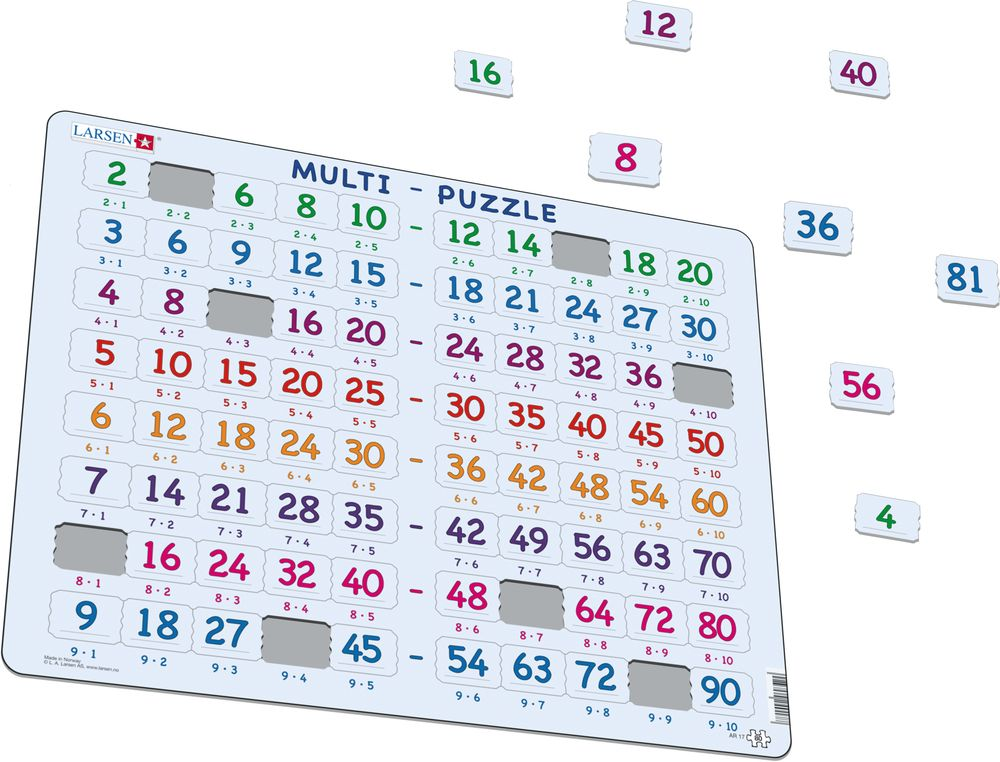# AR17 - Math Puzzle: Multiplication (2 to 9)

## Maxi (36.5x28.5 cm) with 80 pieces

•Learn how to multiply with this educational multiplication puzzle. Learn your 2 to 9 times tables and use the coloured rows of numbers to prompt and guide you. In this puzzle, you will find nine rows of numbers in sequential order. Each row boasts different colour according to the particular number it's being multiplied by. Underneath each puzzle piece, you will find the 'problem' to each answer imprinted on the frame. Conveniently, as the 'problems' are on the frame, the puzzle can also be used as a memory game to prompt the puzzler where to put the correct pieces. This puzzle comes complete with 80 custom-cut pieces and is aimed at slightly older children learning multiplication.

AR17 - Math Puzzle: Multiplication (2 to 9) is available in the following versions:

• Neutral (EAN/GTIN: 7023852117507)